# Problem 57565. Easy Sequences 92: Number of Roots of a System of Linear Diophantine Equations

One of the tasks that Matlab is very good at, is in solving systems of linear equations.
In this problem we shall tackle a system of linear Diophantine Equations in which the roots are limited to certain range.
Given the number of variables n, positive integers, a, b and a root limit, L, create the function, numRoots(n,a,b,L), that outputs the number of posible integer root sets of the following system of equations: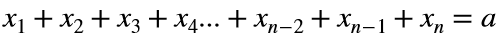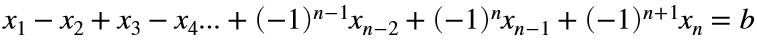with: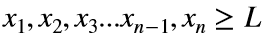.
For example, if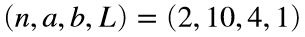, the system of equations: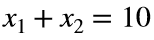and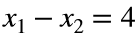, where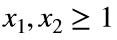, has only one root set, namely: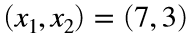. Therefore, numRoots(2,10,4,1) = 1.
If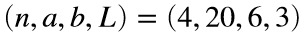, two of the possible roots of: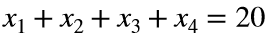; and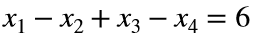where: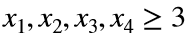are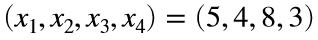and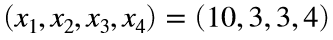. In fact, there are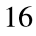possible root sets. Therefore, numRoots(4,20,6,3) = 16.
There are no possible roots for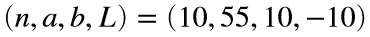and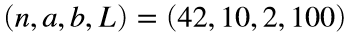, therefore in these cases the function should return: numRoots = 0.
-------------------------
NOTE: As an added challenge, only those solutions with Cody program size of less than or equal to 200 will be accepted.

### Solution Stats

100.0% Correct | 0.0% Incorrect
Last Solution submitted on Feb 08, 2023

### Community Treasure Hunt

Find the treasures in MATLAB Central and discover how the community can help you!

Start Hunting!